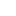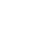# Standardization of 0.1N Sodium hydroxide (NaOH) Against 0.1N Oxalic acid

Standardization of 0.1N Sodium hydroxide (NaOH) Against 0.1N Oxalic acid

#### Theory:

Acid base titration is a method of determining unknown concentration of an acid or a base. It is a quantitative analysis by which the exactly neutralizing amount of other reagent can be determined. It is standardized with a standard solution of base or acid of known concentration.

#### Acid-Base Concepts:

There are many acid base concepts; some of them are given as

#### Arrhenius Concept

Acid

According to Arrhenius concept, Acid is a substance which gives protons in water.

For example

HCl ⇌ H+ +Cl

In this example the HCl is an acid, according to the concept.

Base

According to Arrhenius concept, Base is a substance which gives hydroxyl ions in water.

For example

NaOH ⇌ Na+ + OH

Here sodium hydroxide is a base.

#### Acid

Acid is a substance that can donate proton other substances. For example the specie which carries the positive charge or is electron deficient H+, AlCl3, BF3, etc.

Base

Base is a species which can donate an electron pair to form coordinates covalent bond. It may be a neutral atom or compound or bearing negative charge on it. e.g. NH3, H2O, NH2, OH

: NH3 + BF3→ [H3N—BF3] +

Here ammonia is acting as a base while the BF3 is showing acidic character.

#### Lux-flood concept:

Acid

According to the Lux-flood concept acid is an oxide acceptor.

Base

According to this concept the base is an oxide Ion donor.

CaO + SiO2 → CaSiO3

#### Usanovich concept:

Acid

According to this concept an acid is a chemical species which is capable of combining with anion or giving up cation.

Base

A species that is capable of giving up anions or combining with cation.

SO3 + Na2O → Na2SO4

#### SHAB Concept:

Soft acid

According to this, an acid in which acceptor atom has large size zero or low positive charge and several valence electrons that can be easily excited is known as soft acid. For example Cu+, Ag+, Ti+

Hard acid

An acid is one in which acceptor atom has small size high positive, charge and has no valence electron that can be easily excited.

e.g Li+, Cd+, Al+

Soft base

The specie in which donor atom is of high polarizibility low electronegativity, can easily be oxidized and dissociated with orbital of low energy or rare position of their valence electron easily shifted by positive charge in their neighbor.

e.g, H+, CO, P and I

Hard base

The base in which the donor atom is a of low polarizibility, which cannot be is easily oxidized are associated with empty orbital of high energy and hence hold tightly to their valence electron.

e.g, F, Cl

#### Primary standard solution:

The solution of accurate concentration as stable solution is known as primary standard solution. Oxalic acid once formed cannot be discarded so it is primary standard solution

#### Secondary standard solution:

The solution of inaccurate concentration or unstable solution is called secondary standard solution in this titration NaOH is secondary standard solution

#### Indicator:

Phenolphthalein is used as indicator in most acid base titration reactions. It shows the color changes with changing PH. It is colourless which dissociate to form an ion having pink colour.#### Procedure:

• Glassware was washed, cleaned and fixed accurately and carefully
• Burette was filled up to the mark with standard solution of oxalic acid
• 10 ml of sample solution of sodium hydroxide was taken in the flask and added few drops of phenolphthalein
• Now sodium hydroxide was titrated against the oxalic acid volume of acid used was noted
• Repeated the experiment for three times to take mean accurate reading
• Now by using the formula normality of sodium hydroxide was calculated.

N1V1=N2V2

#### Calculations for solutions preparation:

Preparation of 0.1N NaOH

Equivalent weight of NaOH=40g

S1000ml of 1N solution contain NaOH = 40g

0.1 N solution in 1000 ml contain NaOH = 40×0.1

=4g

So, 4 grams of NaOH was taken and diluted up to 1000 ml of water to prepare 0.1N NaOH

Preparation of 0.1N Oxalic acid

Molecular weight of oxalic acid=90g

Equivalent weight of carboxylic acid = 90/2

=45g

1000 of 1N solution contain oxalic acid = 45g

0.1 N solution in 1000 ml contain oxalic acid=45×0.1

=4.5g

4.5 gram of oxalic acid was taken and diluted to 1000 ml to form 0.1 N oxalic acid solutions.

#### Calculations and observation:

Normality of oxalic acid = 0.1 N

Normality of NaOH =?

 Sr. NO Initial reading Final reading Volume used 1 0 ml 8.6 ml 8.6 ml 2 8.6 ml 17.5 ml 8.9 ml 3 17.5 ml 26.0 ml 8.5 ml

Mean volume =

V1 = 8.6 ml

Acid = Base

N1V1=N2V2

1. × 8.6 = N2 × 10

N2 = 0.086 N

#### Systematic errors and precautions

During the Performance of experiment on number of systematic and individual error may occur such as:

Determinate errors

Indeterminate errors

These errors may disturb and alter the result of experiment. Determinate errors can be minimised by using different techniques and also by extensive care and by finding fault in the construction and buy calibration and use of proper solvent.

#### Results:

Normality of sodium hydroxide is 0.086N

#### Standardization of 0.1N Sodium hydroxide (NaOH) Against 0.1N Oxalic acid1.